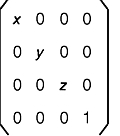[ Previous | Next | Contents | Glossary | Home | Search ]
OpenGL 1.1 for AIX: Reference Manual

## glScale Subroutine

### Purpose

Multiplies the current matrix by a general scaling matrix.

### Library

OpenGL C bindings library: libGL.a

### C Syntax

```void glScaled(GLdouble X,
GLdouble Y,
GLdouble Z)```
```void glScalef(GLfloat X,
GLfloat Y,
GLfloat Z)```

### Parameters

 X, Y, Z Specify scale factors along the X, Y, and Z axes, respectively.

### Description

The glScale subroutine produces a general scaling along the X, Y, and Z axes. The three arguments indicate the desired scale factors along each of the three axes. The resulting matrix is as follows:The current matrix is multiplied by this scale matrix, with the product replacing the current matrix. That is, if M is the current matrix and S is the scale matrix, M is replaced with MS. (See the glMatrixMode subroutine for information on specifying the current matrix.)

If the matrix mode is either GL_MODELVIEW or GL_PROJECTION, all objects drawn after glScale is called are scaled. Use the glPushMatrix and glPopMatrix subroutines to save and restore the unscaled coordinate system.

### Notes

If scale factors other than 1.0 are applied to the modelview matrix and lighting is enabled, automatic normalization of normals should probably also be enabled. (Use the glEnable and glDisable subroutines with the GL_NORMALIZE argument.)

### Errors

 GL_INVALID_OPERATION The glScale subroutine is called between a call to glBegin and the corresponding call to glEnd.

### Associated Gets

Associated gets for the glScale subroutine are as follows. (See the glGet subroutine for more information.)

glGet with argument GL_MATRIX_MODE

glGet with argument GL_MODELVIEW_MATRIX

glGet with argument GL_PROJECTION_MATRIX

glGet with argument GL_TEXTURE_MATRIX.

### Files

 /usr/include/GL/gl.h Contains C language constants, variable type definitions, and ANSI function prototypes for OpenGL.

### Related Information

The glBegin or glEnd subroutine, glEnable subroutine, glMatrixMode subroutine, glMultMatrix subroutine, glPushMatrix subroutine, glRotate subroutine, glTranslate subroutine.

[ Previous | Next | Contents | Glossary | Home | Search ]